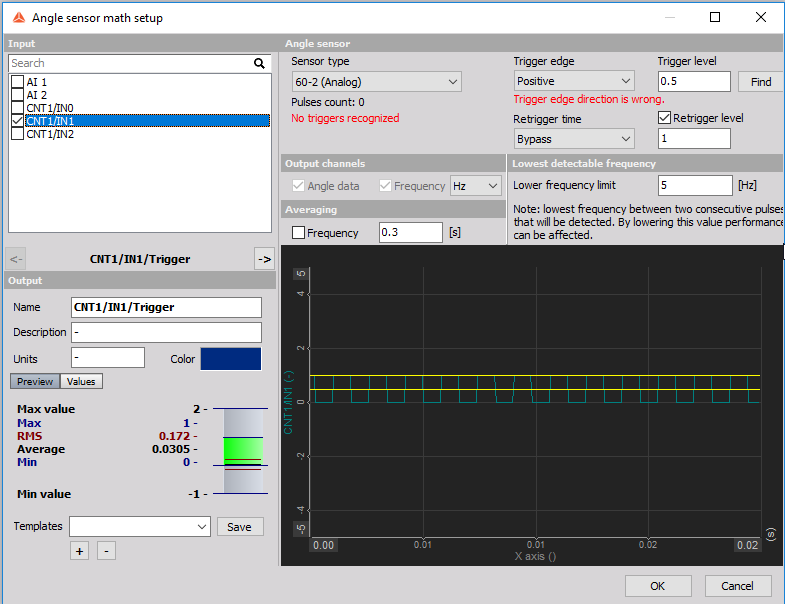It is possible to use three single counter signals with only one counter connector. You can connect each counter to a separate "IN" pin and to the GND pin. As both inputs are connected to the same ground, you must consider the counter potentials, as inputs are not "isolated" in this case.You can only use one input as a counter, with the "super-counter" functionality. Than choose the sensor type with only one signal input, but set other DI  channels to "used".Then use the other DI channels in "Analog sensor math" where the counter channel will be calculated ( frequency, angle). The inputs which are used in the angle sensor math do not use the super counter functionality.The sensor Angle math uses the selected dynamic acquisition sample rate, which is significantly lower then super counter sampling rate. Due to lower sampling rate, high frequency signal measurements can be incorrectly calculated.